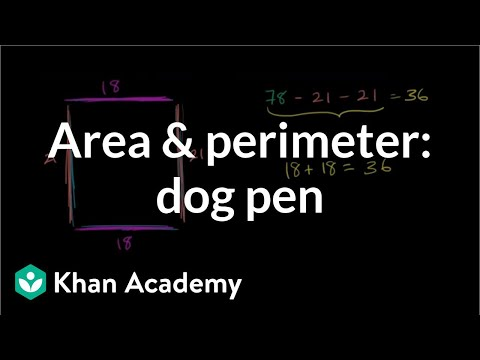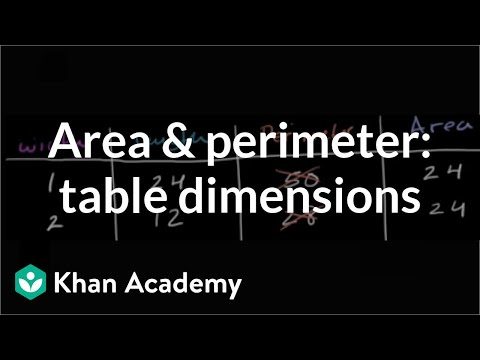Video

# Counting unit squares to find area formula (Full video)

Description: An short lesson on using unit squares to see why multiplying side-lengths can also find the area of rectangles And in fact, this one right here has the same height and width, so this is actually a square. And since we're doing everything in terms of meters, since all of the dimensions are in meters, I'm going to measure the area in terms of square meters. So let's see how many square meters I can fit onto this yellow rectangle without going outside of its boundary and without overlapping.

### Other videos you might be interested in### Area & perimeter word problem: dog pen (Full video)